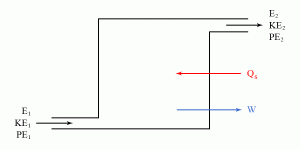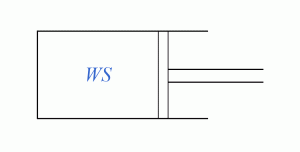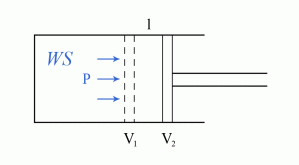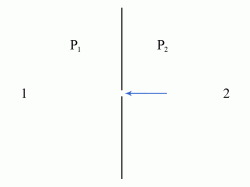•http://facebook.com/
•https://www.google.com/accounts/o8/id
•https://me.yahoo.com

# First Law of Thermodynamics

An introduction to the first law of thermodynamics, and discussing the general energy equation and its application to particular cases.

## Overview

Definitions

Potential energy (PE) is the energy possessed by a quantity of matter by virtue of its position above some specified datum.

Kinetic energy (KE) is the energy possessed by a quantity of matter by virtue of its translational velocity.

Internal energy is the sum of the molecular KE (translational and rotational) and the PE of individual molecules.

Heat engine is any machine that can convert heat into work, and vice versa.

Working substance (WS). The WS is used as the carrier for heat energy. The heat engine carries out the conversion process by a series of changes of state of the WS. The state of the WS is defined by the values of its properties, e.g. pressure, volume, temperature, internal energy, enthalpy. These properties are also sometimes called functions of state.

<br/>

Key facts

The first law of thermodynamics states that energy can neither be created nor destroyed, but only transformed from one form (e.g. work, heat, KE, PE, internal energy) to another.

The first law of thermodynamics can be used to develop the general energy equation, which can be written as:

where , , , and , , are the energies, and functions of state respectively characterizing the first state, , , , and , , are the energies, and functions of state respectively characterizing the second state, is the heat supplied to the WS, and the work done by the WS.

The general energy equation can also be expressed in terms of enthalpies as:

where is the enthalpy characterizing the first state, while is the enthalpy characterizing the second state.

The form of the general energy equation can be adapted to particular cases. For example, for constant volume processes it can be reduced to:

for constant pressure non-flow processes:

for constant pressure flow processes:

for adiabatic non-flow processes:

for adiabatic flow processes:

while for throttling processes it states that there is no change in enthalpy:

The first law of thermodynamics, also sometimes called the law of conservation of energy, states that energy can neither be created nor destroyed, but only transformed from one form to another. The different interchangeable forms of energy include, among others, work, heat, potential energy (PE), kinetic energy (KE), or internal energy.

## The General Energy Equation

The first law of thermodynamics can be used to develop the so-called general energy equation for open systems. Imagine a heat engine taking in a working substance (WS) in an initial state characterized by the energies , , and (see Figure 1).Figure 1 The WS passes through the machine having units of heat supplied to it and giving a work output of units. The WS then leaves the machine with energies , , and .

The work done to drive one unit mass of air through the system, this time diagramed as in Figure 2, is given by:

which can also be written, by taking into account that the force acting on a piston is , as:

where is the pressure and the volume.

Applying the first law of thermodynamics, which states that the energy that enters the system equals that which leaves it, and also taking into account (3), we can write that:

Equation (4) can also be written as:

and is called the general energy equation.

The term is called enthalpy and is denoted with . Thus, the general energy equation can also be written as:

where is the enthalpy characterizing the first state, while is the enthalpy characterizing the second state.

The form of the general energy equation can also be adapted to particular applications. For example, for a petrol engine, the and terms are negligible. Thus equation (6) becomes:

from which we have that:

Therefore, in this case, the heat supplied to the WS equals the work done by the WS plus the gain in enthalpy.

For a gas turbine, we can ignore only the terms, and thus equation (6) becomes:

from which:

Therefore, in this case, the heat supplied to the WS equals the work done by the WS plus the gain in kinetic energy plus the gain in enthalpy.

For a non-flow process, there are no , , or terms. Thus, in this case, equation (5) becomes:

from which:

Hence, the heat supplied to the WS equals the work done by the WS plus the gain in internal energy.

## The Application Of The First Law To Particular Cases

As we previously saw, the first law of thermodynamics can be expressed in terms of the general energy equation (see equations 5 and 6). Also as previously noted, the form of the general energy equation can be adapted to particular applications (see equations 7, 9, and 11). We can further apply the general energy equation to more comprehensive particular cases.

1) Constant Volume Processes

In this case, the work output is zero, and the processes are invariably non-flow.Figure 3 Nearly all the terms in the general energy equation (5) are eliminated, leaving:

or:

Therefore, for constant volume processes, all the heat supplied goes into increasing the internal energy of the WS.

2) Constant Pressure Processes

a) Non-Flow Processes

Consider that the WS experiences an isobaric change in volume as diagramed in Figure 4.Figure 4 As the force acting on the piston is given by:

the work done:

becomes:

or, furthermore:

In the general energy equation (5) most terms are eliminated, leaving:

Taking into account (18), equation (20) becomes:

or, furthermore:

Thus:

Therefore, for constant pressure non-flow processes, the heat supplied to the WS equals the gain in enthalpy.

b) Flow Processes

In this case, considering that all the changes to the kinetic and potential energies are negligible, the general energy equation (6) becomes:

However, it is impossible to devise a machine that operates a constant pressure flow process giving a constant work output. Therefore, in all practical cases, the heat supplied to the WS equals the gain in enthalpy.

These are processes during which there is no heat transfer between the WS and the surroundings.

a) Non-Flow Processes

For adiabatic non-flow processes, the general energy equation (5) becomes:

Therefore, the work done is:

and it thus equivalates the loss of internal energy.

b) Flow Processes

This category includes all rotary turbines and compressors. The general energy equation (6) can be written in this case as:

from which the work done becomes:

and it thus equivalates the loss of enthalpy.

4) Throttling Processes

Consider two points, and , which are far enough from the orifice so that the terms are negligible (see Figure 5).Figure 5 Furthermore, consider that there is no heat supplied and there is no work output. Therefore, the general energy equation (6) becomes in this case:

Hence, for throttling processes, there is no change in enthalpy.

Example:
[imperial]
##### Example - Horsepower of a gas turbine
Problem
Twenty pounds of gas flow per second through a gas turbine. The inlet pressure is (), the specific volume is , and the inlet velocity is . In passing through the engine, the internal energy drops by , while are lost through radiation. If the gas is discharged at and at with a specific volume of , find the horsepower () developed by the turbine.
Workings
From the general energy equation we can write that:

which gives:

In order to calculate from (2) we have to analyze each individual term. From the hypothesis we know that:

Still from the hypothesis, and also considering that , and , the term expressed in can be calculated as:

from which we obtain:

Following a similar reasoning, the term can be expressed in as:

where is the standard gravity expressed in imperial:

From equation (6) we thus obtain that:

Considering that are lost through radiation, and that the flow rate of the turbine is , the term can be expressed in as:

from which:

By using (3), (5), (8), and (10) in equation (2), we can calculate as:

from which we obtain:

Taking into account that the flow rate of the turbine is , that , that , and also considering (12), we get the horsepower output of the turbine as:
Solution

Last Modified: 14 Oct 11 @ 17:28     Page Rendered: 2022-03-14 16:01:12